Find two equivalent fractions for each fraction.

Example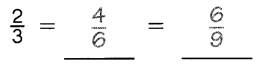Question 1.
$$\frac{3}{4}$$ = ___ = ____

Question 2.
$$\frac{2}{5}$$ = ___ = ____

Question 3.
$$\frac{5}{6}$$ = ___ = ____

Question 4.
$$\frac{1}{7}$$ = ___ = ____

Express each fraction in simplest form.

Question 5.
$$\frac{6}{8}$$ = ___

Question 6.
$$\frac{8}{20}$$ = ___

Question 7.
$$\frac{10}{15}$$ = ___

Question 8.
$$\frac{9}{21}$$ = ___

Rewrite each pair of unlike fractions as like fractions.

Example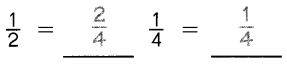Question 9.
$$\frac{1}{4}$$ = ___ $$\frac{5}{12}$$ = ___

Question 10.
$$\frac{1}{10}$$ = ___ $$\frac{2}{5}$$ = ___

Question 11.
$$\frac{5}{9}$$ = ___ $$\frac{2}{3}$$ = ___

Question 12.
$$\frac{3}{8}$$ = ___ $$\frac{9}{16}$$ = ___

Write equivalent fractions for each fraction. Then find the least common denominator of the fractions.

Example
$$\frac{1}{2}$$ = $$\frac{2}{4}$$ = $$\frac{3}{6}$$
$$\frac{2}{3}$$ = $$\frac{4}{6}$$
The least common denominator is 6.

Question 13.
$$\frac{2}{3}$$ =
$$\frac{3}{4}$$ =
The least common denominator is ____

Question 14.
$$\frac{1}{4}$$ =
$$\frac{5}{6}$$ =
The least common denominator is ____

Question 15.
$$\frac{5}{6}$$ = ____
$$\frac{3}{8}$$ = ____
The least common denominator is ____

Shade and label each model to show the tractions. Then complete the addition sentence.

Example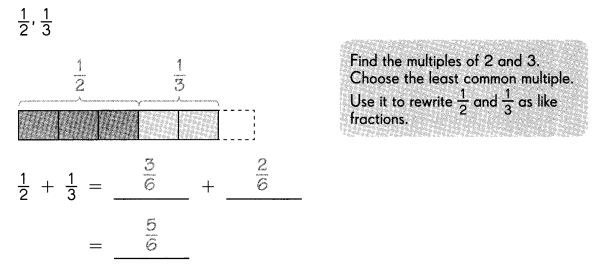Question 16.
$$\frac{1}{5}$$, $$\frac{1}{2}$$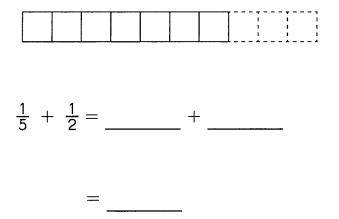Look at the model. Write two addition sentences.

Question 17.
$$\frac{1}{6}$$, $$\frac{1}{4}$$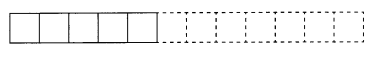$$\frac{1}{6}$$ + $$\frac{1}{4}$$ = ___ + ___
= ____

Question 18.
$$\frac{1}{5}$$, $$\frac{2}{3}$$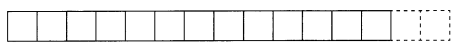$$\frac{1}{5}$$ + $$\frac{2}{3}$$ = ____ + ___
= ____

Question 19.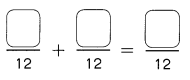Question 20.
Addition sentence 2 (fractions in simplest form):
____ + ____ = ____

Add. Express each sum in simplest form.

Question 21.
$$\frac{1}{3}$$ + $$\frac{1}{9}$$ =

Question 22.
$$\frac{5}{8}$$ + $$\frac{2}{4}$$ =

Question 23.
$$\frac{1}{2}$$ + $$\frac{6}{7}$$ =

Question 24.
$$\frac{4}{8}$$ + $$\frac{1}{5}$$ =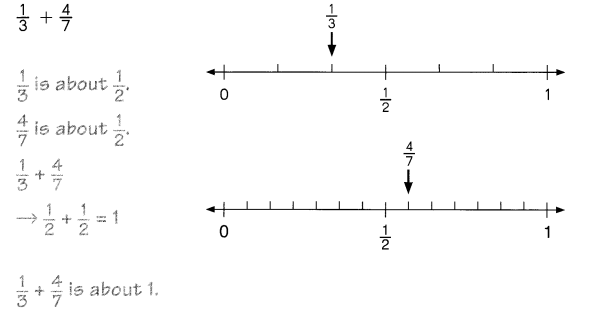$$\frac{2}{3}$$ + $$\frac{2}{9}$$
$$\frac{7}{9}$$ + $$\frac{1}{7}$$ + $$\frac{3}{5}$$